HashMap底层红黑树实现(自己实现一个简单的红黑树)

+关注继续查看

JDK集合源码之HashMap解析(上)

JDK集合源码之HashMap解析(下)

1.树结构入门

1.1 什么是树？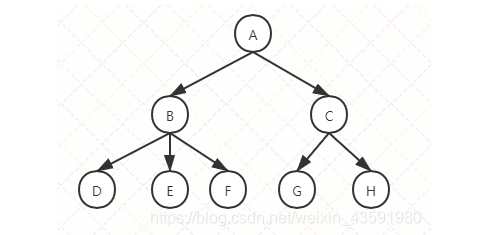①、节点：上图的圆圈，比如A,B,C等都是表示节点。节点一般代表一些实体，在java面向对象编程中，节点一般代表对象。

②、边：连接节点的线称为边，边表示节点的关联关系。一般从一个节点到另一个节点的唯一方法就是沿着一条顺着有边的道路前进。在Java当中通常表示引用。

1.2 树结构常用术语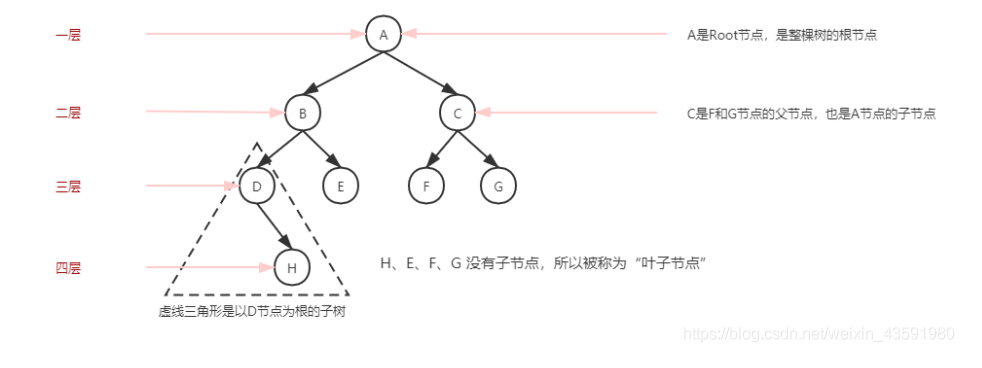①、路径：顺着节点的边从一个节点走到另一个节点，所经过的节点的顺序排列就称为“路径”。

②、根：树顶端的节点称为根。一棵树只有一个根，如果要把一个节点和边的集合称为树，那么从根到其他任何一个节点都必须有且只有一条路径。A是根节点。

③、父节点：若一个节点含有子节点，则这个节点称为其子节点的父节点

④、子节点：一个节点含有的子树的节点称为该节点的子节点；F、G是C节点的子节点。

⑤、兄弟节点：具有相同父节点的节点互称为兄弟节点；F、G节点互为兄弟节点。

⑥、叶节点：没有子节点的节点称为叶节点，也叫叶子节点，比如上图的H、E、F、G都是叶子节点。

⑦、子树：每个节点都可以作为子树的根，它和它所有的子节点、子节点的子节点等都包含在子树中。

⑧、节点的层次：从根开始定义，根为第一层，根的子节点为第二层，以此类推。

⑨、深度：对于任意节点n,n的深度为从根到n的唯一路径长，根的深度为0；（从上往下看）

⑩、高度：对于任意节点n,n的高度为从n到一片树叶的最长路径长，所有树叶的高度为0；（从下往上看）

1.3 二叉搜索树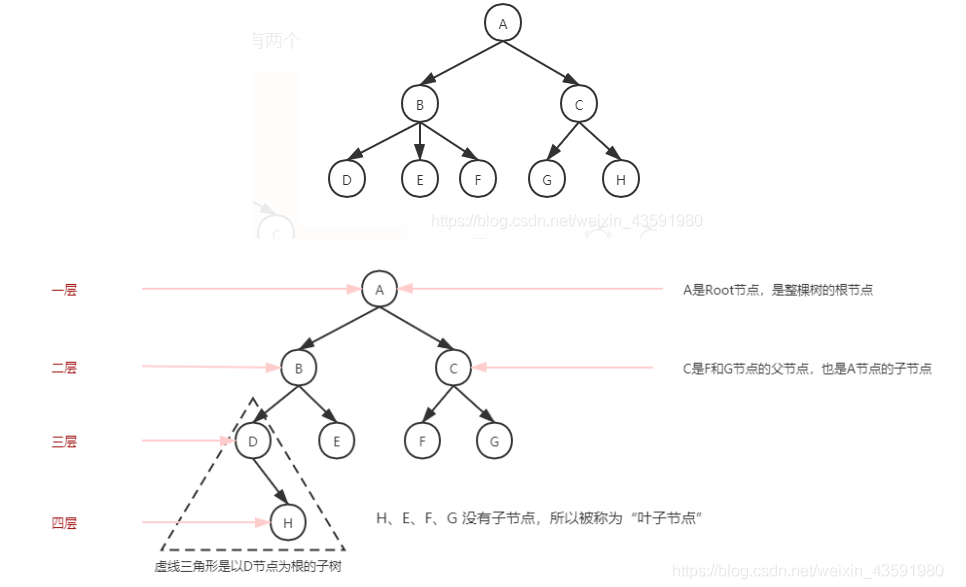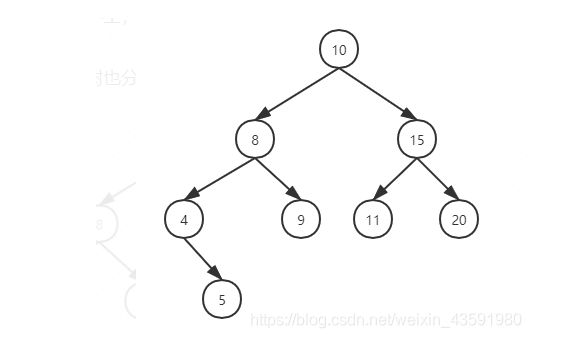①、查找值比当前节点值大，则搜索右子树；

②、查找值等于当前节点值，停止搜索（终止条件）；

③、查找值小于当前节点值，则搜索左子树；

①、中序遍历:左子树——》根节点——》右子树

②、前序遍历:根节点——》左子树——》右子树

③、后序遍历:左子树——》右子树——》根节点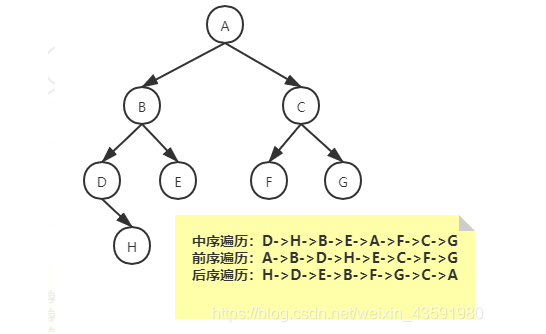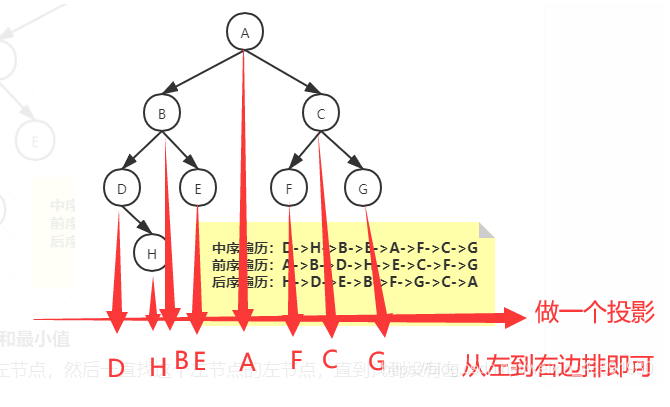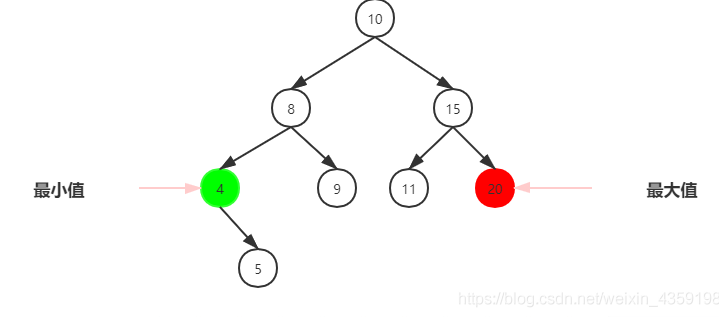1、该节点是叶节点（没有子节点）

2、该节点有一个子节点

3、该节点有两个子节点

①、删除没有子节点的节点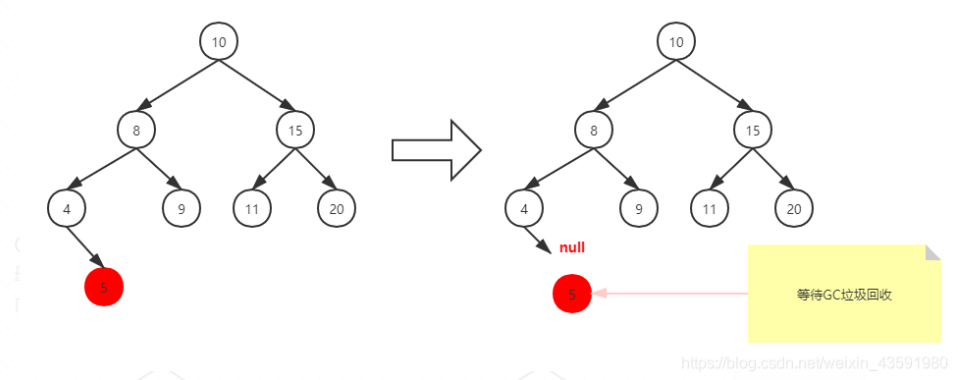②、删除有一个子节点的节点

③、删除有两个子节点的节点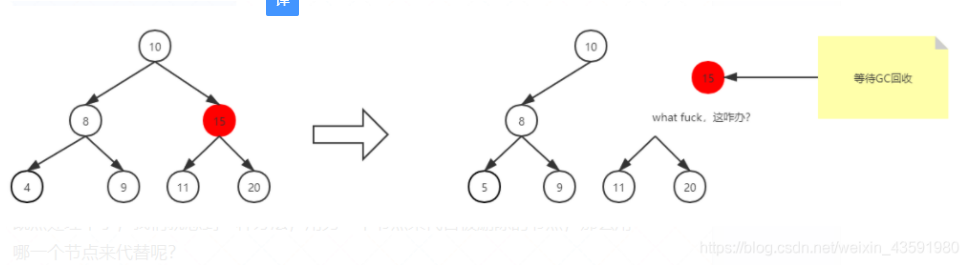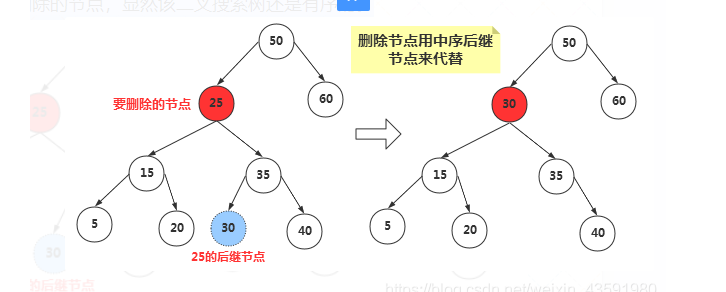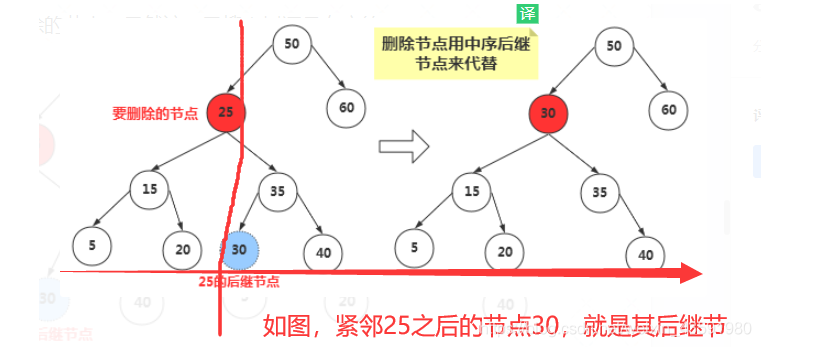④、删除有必要吗？

1.回顾经典-二分查找算法

[1,2,3,4,5,6,7,8,9。。。。。。。100]

@Test
public void test03() {
int[] arr = new int[]{1,2,3,4,5,6,7,8,9,10};
System.out.println(binarySearch(arr,3));
}
/*
* 二分查找
* @param: arr
* @param: data
* @return: int
* @create: 2020/11/6 13:29
* @author: csp1999
*/
public static int binarySearch(int[] arr, int data) {
int low = 0;
int height = arr.length - 1;
while (low <= height) {
int mid = low + (height - low) / 2;
if (arr[mid] < data) {
low = mid + 1;
} else if (arr[mid] == data) {
return mid;
} else {
height = mid - 1;
}
}
return -1;
}

2.二分查找算法最大的缺陷是什么？

3.数组有什么缺陷？

4.那怎么才能拥有二分查找的高性能又能拥有链表一样的灵活性？

5.二分查找算法时间复杂度推算过程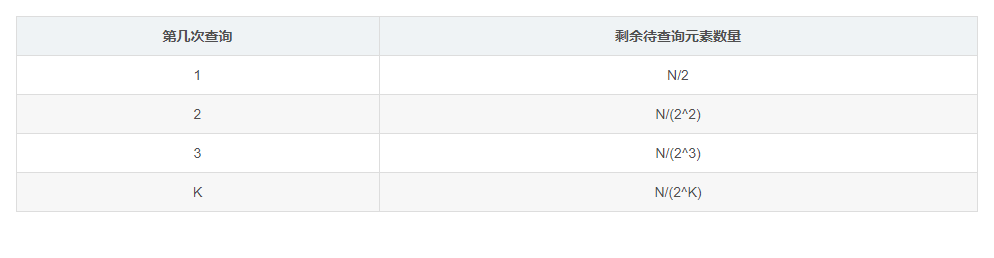N/(2^K)=1 => 2^K = N => K = log2 (N) => 二分查找算法时间复杂度：O(log2(N)) => O(logN)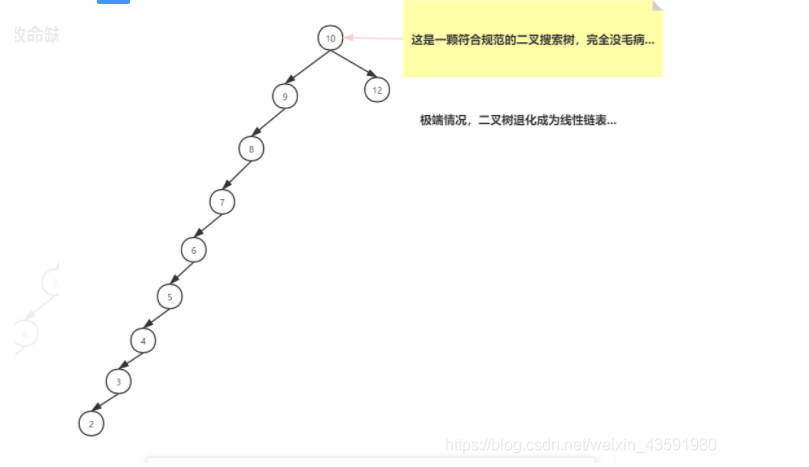O(N)

AVL树简介：

AVL树有什么特点？

1、具有二叉查找树的全部特性。

2、每个节点的左子树和右子树的高度差至多等于1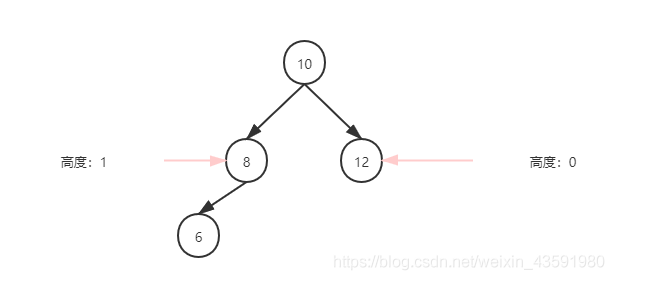2.红黑树原理讲解

|—红黑树的性质

|—红黑树有几种变化策略？（为满足红黑树性质）

|—改变颜色

|—左旋

|—右旋

|—红黑树的查找

|—红黑树的插入

|—情景1：红黑树为空树

|—情景2：插入节点的key已经存在

|—情景3：插入节点的父节点为黑色

|—情景4：插入节点的父节点为红色

|—情景4.1：叔叔节点存在，并且为红色（父-叔 双红）

|—情景4.2：叔叔节点不存在，或者为黑色，父节点为爷爷节点的左子树

|—情景4.2.1：插入节点为其父节点的左子节点（LL情况）

|—情景4.2.2：插入节点为其父节点的右子节点（LR情况）

|—情景4.3：叔叔节点不存在，或者为黑色，父节点为爷爷节点的右子树

|—情景4.3.1：插入节点为其父节点的右子节点（RR情况）

|—情景4.3.2：插入节点为其父节点的左子节点（RL情况）

|—红黑树插入案例分析

2.1 红黑树的性质：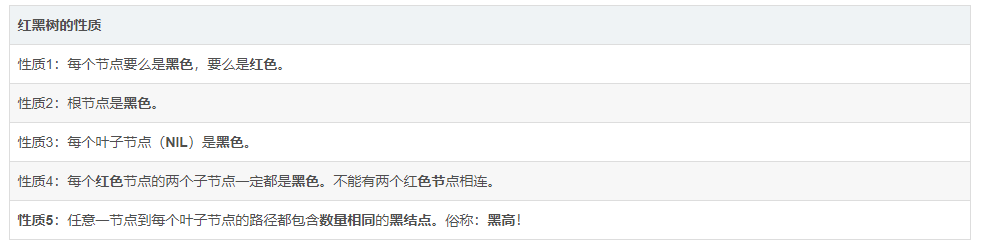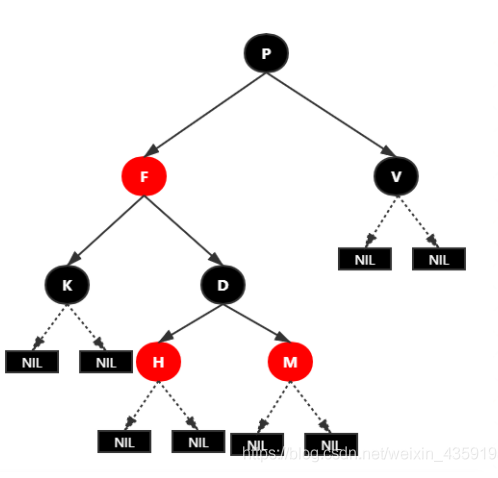**1.变色：**结点的颜色由红变黑或由黑变红。

**2.左旋：**以某个结点作为支点(旋转结点)，其右子结点变为旋转结点的父结点，右子结点的左子结点变为旋转结点的右子结点，左子结点保持不变。

**3.右旋：**以某个结点作为支点(旋转结点)，其左子结点变为旋转结点的父结点，左子结点的右子结点变为旋转结点的左子结点，右子结点保持不变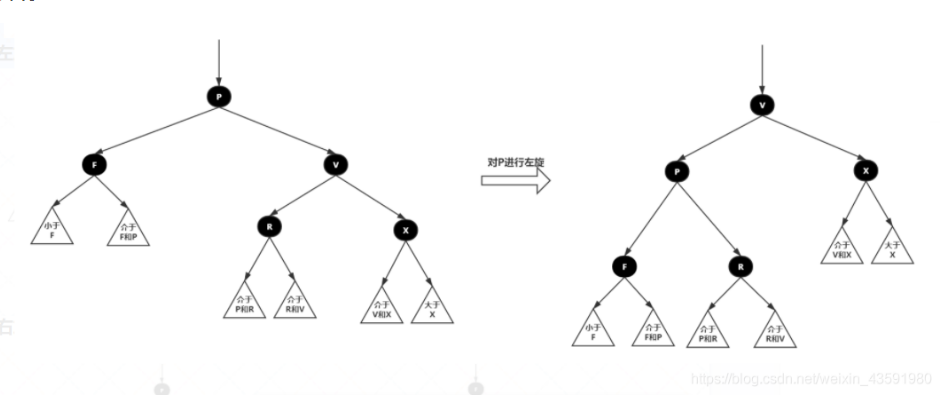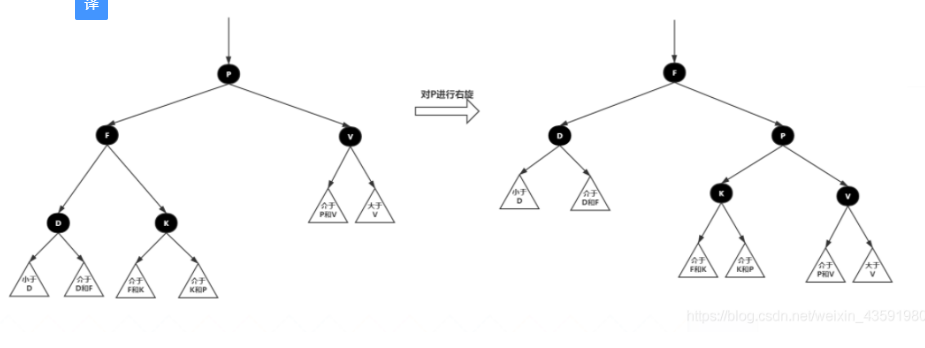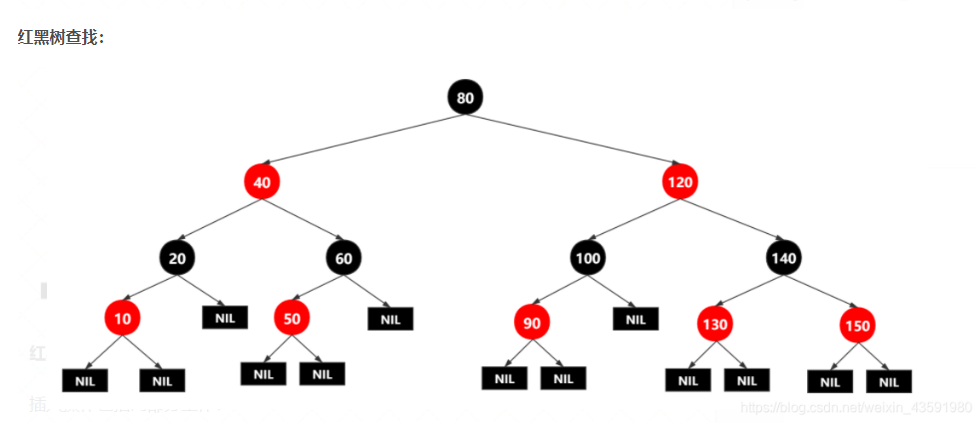1.查找插入的位置

2.插入后自平衡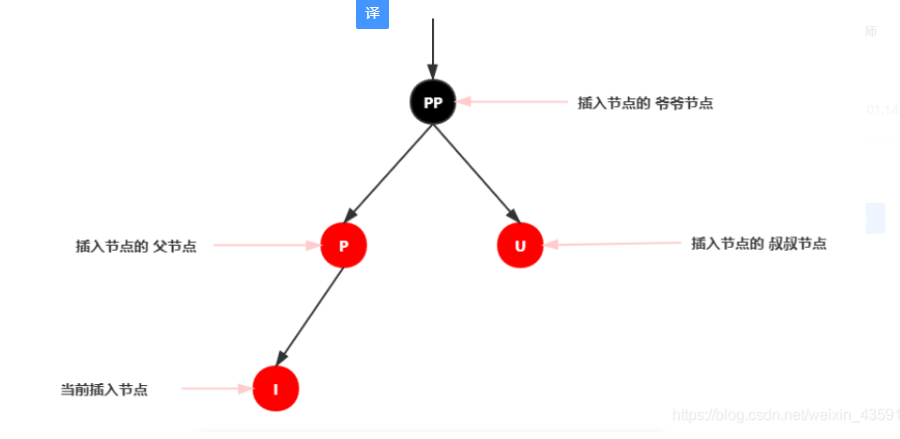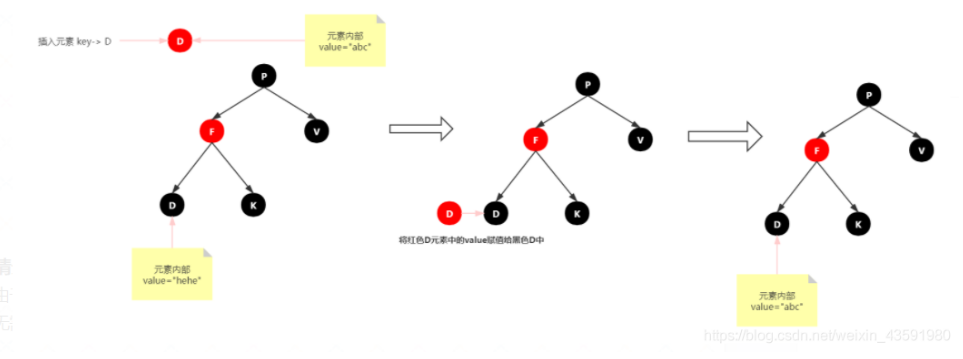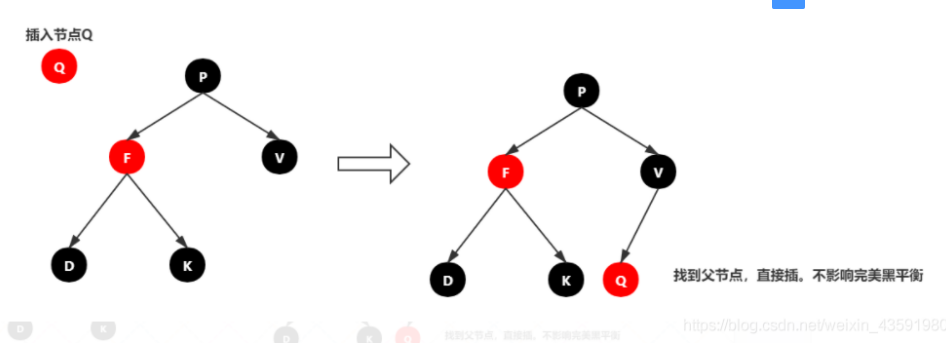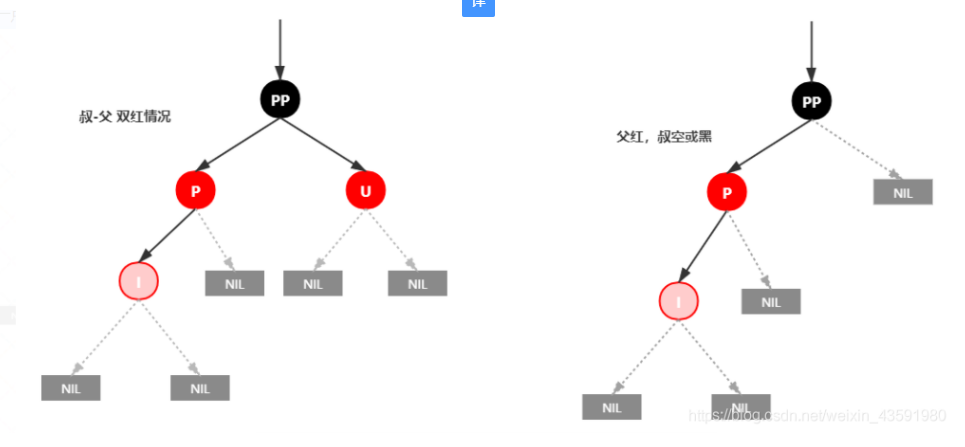1.将P和U节点改为黑色

2.将PP改为红色

3.将PP设置为当前节点，进行后续处理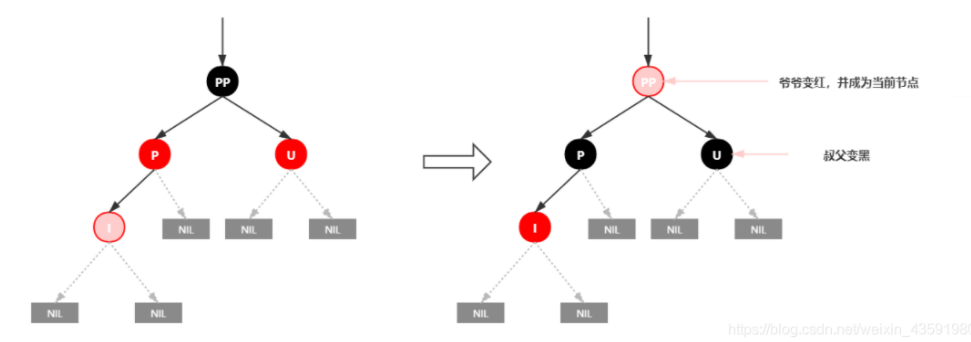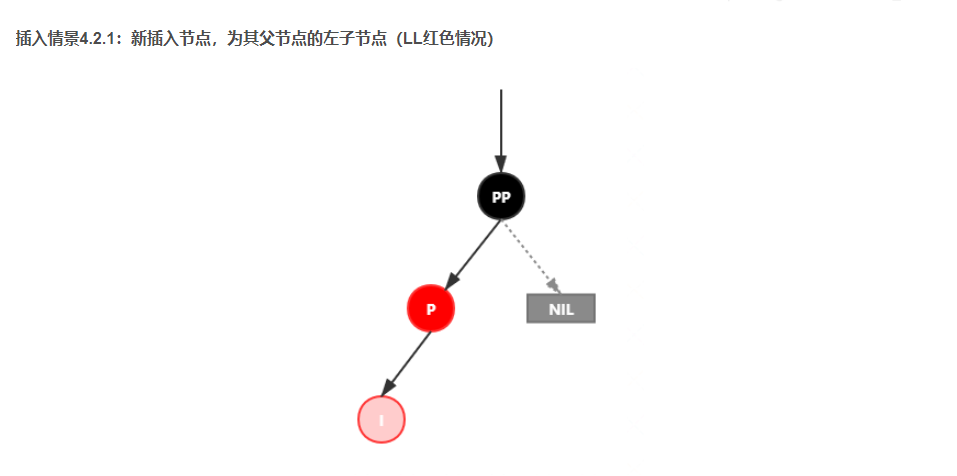1.变颜色：将P设置为黑色，将PP设置为红色

2.对PP节点进行右旋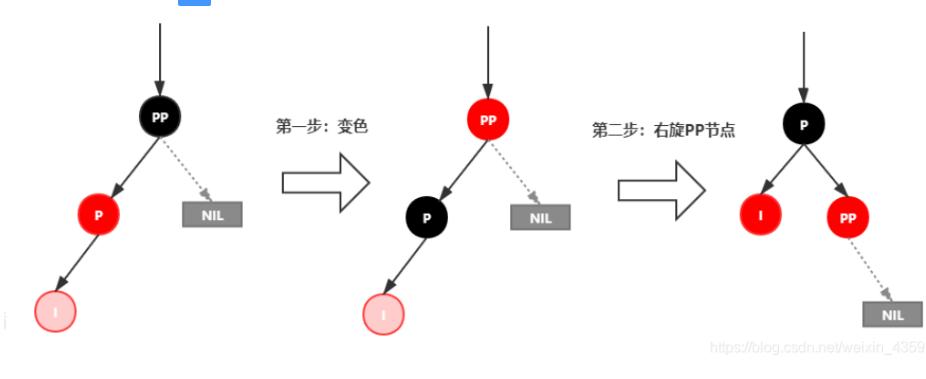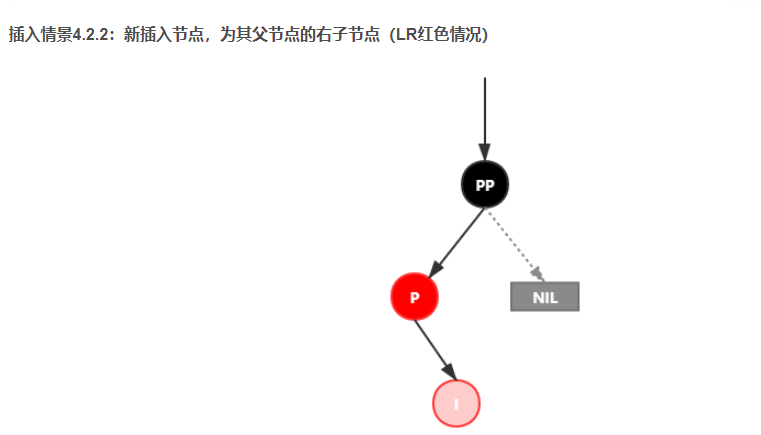1.对P进行左旋

2.将P设置为当前节点，得到LL红色情况

3.按照LL红色情况处理（1.变颜色 2.右旋PP）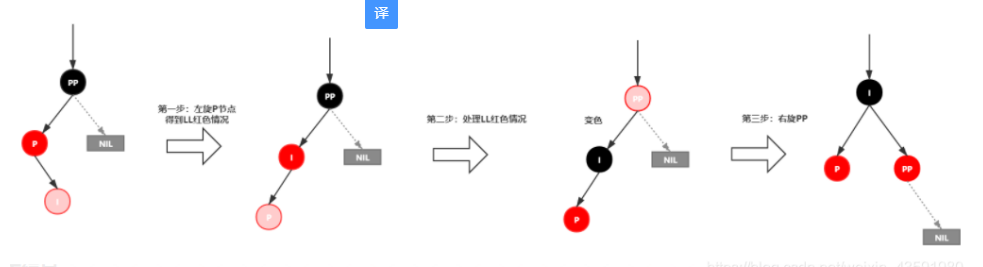*插入情景4.3：**叔叔结点不存在或为黑结点，并且插入结点的父亲结点是祖父结点的右子结点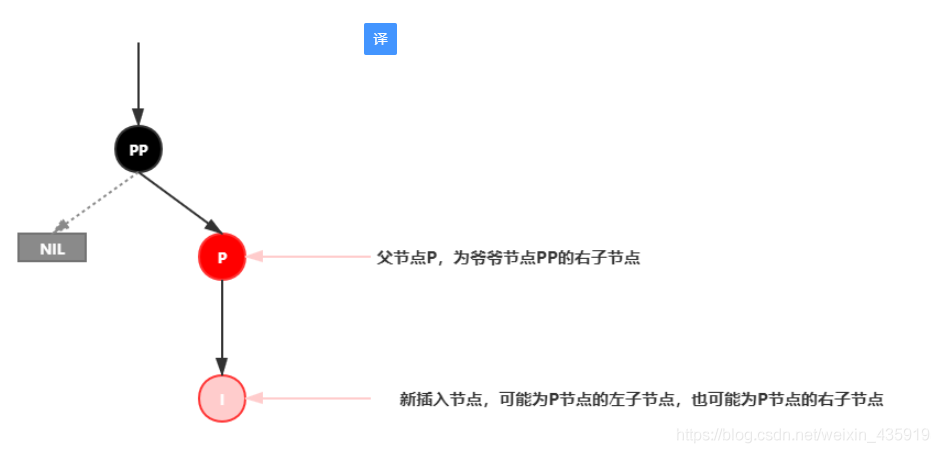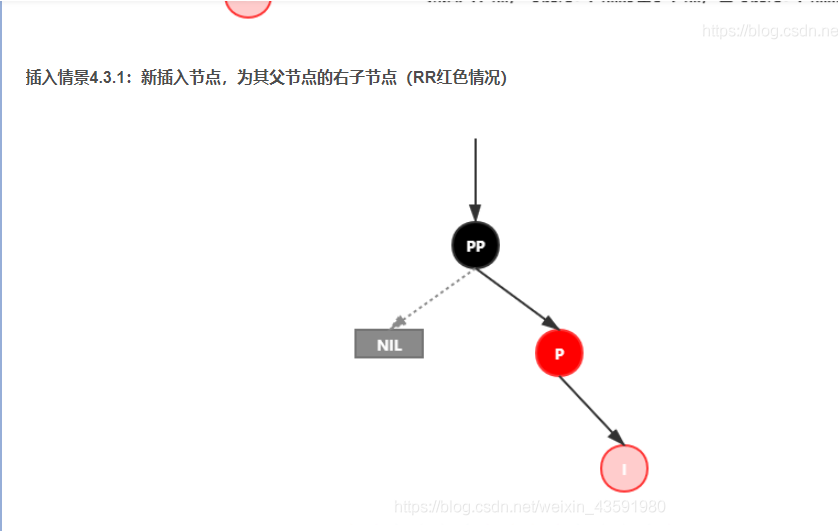1.变颜色：将P设置为黑色，将PP设置为红色

2.对PP节点进行左旋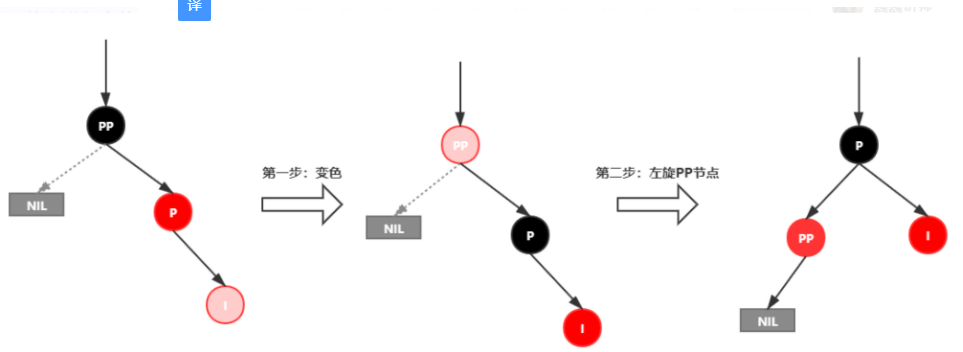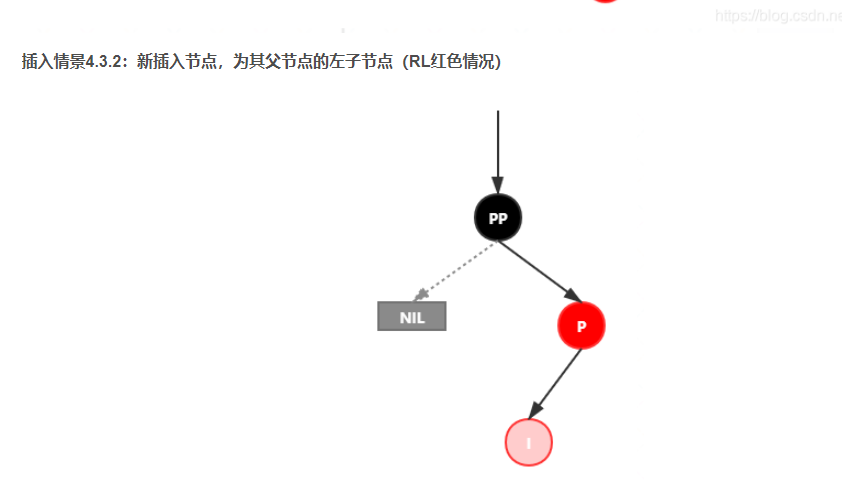1.对P进行右旋

2.将P设置为当前节点，得到RR红色情况

3.按照RR红色情况处理（1.变颜色 2.左旋PP）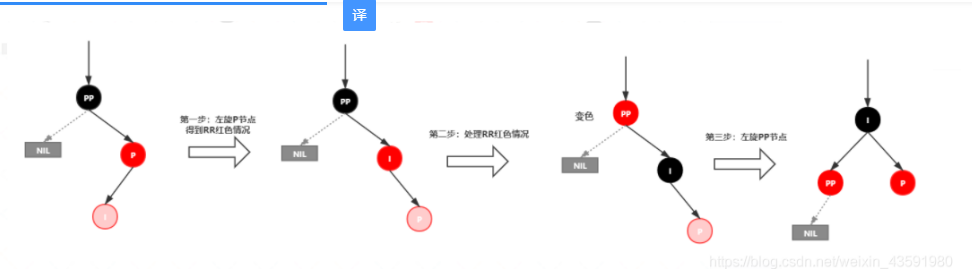2.2 红黑树案例分析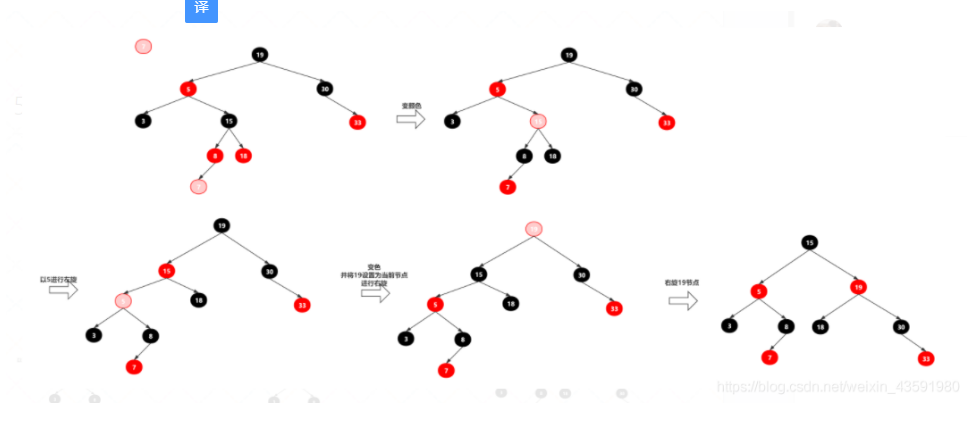3.手写红黑树

①创建RBTree，定义颜色

②创建RBNode

③辅助方法定义：parentOf(node)，isRed(node)，setRed(node)，setBlack(node)，inOrderPrint()

④左旋方法定义：leftRotate(node)

⑤右旋方法定义：rightRotate(node)

⑥公开插入接口方法定义：insert(K key, V value);

⑦内部插入接口方法定义：insert(RBNode node);

⑧修正插入导致红黑树失衡的方法定义：insertFIxUp(RBNode node);

⑨测试红黑树正确性

RBTree.java

package com.haust.map;

/**
* @Auther: csp1999
* @Date: 2020/11/06/18:00
* @Description: ①创建RBTree，定义颜色
* <p>
* ②创建RBNode
* <p>
* ③辅助方法定义：parentOf(node)，isRed(node)，setRed(node)，setBlack(node)，inOrderPrint()
* <p>
* ④左旋方法定义：leftRotate(node)
* <p>
* ⑤右旋方法定义：rightRotate(node)
* <p>
* ⑥公开插入接口方法定义：insert(K key, V value);
* <p>
* ⑦内部插入接口方法定义：insert(RBNode node);
* <p>
* ⑧修正插入导致红黑树失衡的方法定义：insertFIxUp(RBNode node);
* <p>
* ⑨测试红黑树正确性
*/
public class RBTree<K extends Comparable<K>, V> {
private static final boolean RED = true;// 红
private static final boolean BLACK = false;// 黑
/**
* 树根的引用
**/
private RBNode root;

public RBNode getRoot() {
return root;
}

/**
* 获取当前节点的父节点
*
* @param node
* @return
*/
private RBNode parentOf(RBNode node) {
if (node != null) {
return node.parent;
}
return null;
}

/**
* 节点是否为红色
*
* @param node
* @return
*/
private boolean isRed(RBNode node) {
if (node != null) {
return node.color == RED;
}
return false;
}

/**
* 节点是否为黑色
*
* @param node
* @return
*/
private boolean isBlack(RBNode node) {
if (node != null) {
return node.color == BLACK;
}
return false;
}

/**
* 设置节点为红色
*
* @param node
*/
private void setRed(RBNode node) {
if (node != null) {
node.color = RED;
}
}

/**
* 设置节点为黑色
*
* @param node
*/
private void setBlack(RBNode node) {
if (node != null) {
node.color = BLACK;
}
}

/**
* 中序打印二叉树
*/
public void inOrderPrint() {
inOrderPrint(this.root);
}

private void inOrderPrint(RBNode node) {
if (node != null) {
inOrderPrint(node.left);
System.out.println("key:" + node.key + ",value:" + node.value);
inOrderPrint(node.right);
}
}

/**
* 左旋方法
* 左旋示意图：左旋x节点
*   p                   p
*   |                   |
*   x                   y
*  / \      ---->      / \
* lx  y               x   ry
*    / \             / \
*   ly  ry          lx  ly
*
* 左旋做了几件事？
* 1.将x的右子节点指向y的左子节点(ly)，并且把y的左子节点更新为x
* 2.当x的父节点(不为空时)，更新y的父节点为x的父节点，并将x的父节点 指定 子树(当前x的子树位置) 指定为y
* 3.将x的父节点更新为y，将y的左子节点更新为x
*/
private void leftRotate(RBNode x) {
RBNode y = x.right;// 获得y
// 1.将x的右子节点指向y的左子节点(ly)，并且把y的左子节点更新为x
x.right = y.left;
if (y.left != null) {
y.left.parent = x;
}

// 2.当x的父节点(不为空时)，更新y的父节点为x的父节点，并将x的父节点 指定 子树(当前x的子树位置) 指定为y
if (x.parent != null) {
y.parent = x.parent;
if (x == x.parent.left) {// 如果x是其父节点的左子节点，则将y放在x父节点的左边
x.parent.left = y;
} else {
x.parent.right = y;// 如果x是其父节点的右子节点，则将y放在x父节点的右边
}
} else {// 说明x为根节点，此时需要更新y为根节点 的引用
this.root = y;
this.root.parent = null;// 根节点无父节点
}

// 3.将x的父节点更新为y，将y的左子节点更新为x
x.parent = y;
y.left = x;
}

/**
* 右旋方法
* 右旋示意图：右旋y节点
*
*     p                       p
*     |                       |
*     y                       x
*    / \          ---->      / \
*   x   ry                  lx  y
*  / \                         / \
* lx  ly                      ly  ry
*
* 右旋都做了几件事？
* 1.将y的左子节点指向x的右子节点，并且更新x的右子节点的父节点为y
* 2.当y的父节点不为空时，更新x的父节点为y的父节点，更新y的父节点的指定子节点（y当前位置） 为x
* 3.更新y 的父节点为x ,更新x 的右子节点为y
*/
private void rightRotate(RBNode y) {
RBNode x = y.left;// 获得 x
// 1.将x的右子节点 赋值 给了 y 的左子节点，并且更新x的右子节点的父节点为 y
y.left = x.right;
if(x.right != null) {
x.right.parent = y;
}

// 2.将y的父节点p（非空时）赋值给x的父节点，同时更新p的子节点为x（左或右）
if(y.parent != null) {
x.parent = y.parent;
if(y.parent.left == y) {// 如果y是其父节点的左子节点，则将x放在y父节点的左边
y.parent.left = x;
} else {// 如果y是其父节点的右子节点，则将x放在y父节点的右边
y.parent.right = x;
}
} else {// 说明y为根节点，此时需要更新x为根节点 的引用
this.root = x;
this.root.parent = null;// 根节点无父节点
}

// 3.将x的右子节点赋值为y，将y的父节点设置为x
x.right = y;
y.parent = x;
}

/**
* public插入方法
*
* @param key
* @param value
*/
public void insert(K key, V value) {
RBNode node = new RBNode<>();
node.setKey(key);
node.setValue(value);
// 新节点 一定要是红色！
node.setColor(RED);

insert(node);
}

private void insert(RBNode node) {
// 第一步：查找当前要插入节点node的父节点
RBNode parent = null;// 声明要插入节点node的父节点
RBNode x = this.root;

while (x != null) {
parent = x;

/**
* cmp > 0 说明node.key 大于 x.key 需要到x 的右子树查找
* cmp == 0 说明node.key 等于 x.key 需要进行替换操作
* cmp < 0 说明node.key 小于 x.key 需要到x 的左子树查找
*/
int cmp = node.key.compareTo(x.key);
if (cmp > 0) {
x = x.right;
} else if (cmp == 0) {
x.setValue(node.getValue());
return;// 修改完后 就不再继续往下面的代码执行了
} else {
x = x.left;
}
}

/**
* 退出上面的while循环后，到这里，说明树中没有相同key 的元素
*
* 需要添加新元素node到 x(parent) 目前位置的左子树/右子树
*/
node.parent = parent;
if (parent != null) {
// 判断node与parent 的key 谁大
int cmp = node.key.compareTo(parent.key);
if (cmp > 0) {// 当前node的key比parent 的key大，需要把node放入parent 的右子节点
parent.right = node;
} else {// 当前node的key比parent 的key小，需要把node放入parent 的左子节点
parent.left = node;
}
} else {// parent == null; 说明为空树
this.root = node;// 直接给树根赋值为node
}

// 新元素node 加入树中之后，要调用修复红黑树平衡的方法
insertFixUp(node);
}

/**
* 插入后修复红黑树平衡的方法
* |---情景1：如果红黑树为空树,需要将根节点染为黑色
* |---情景2：如果插入节点的key已经存在,(这种情况不需要处理,因为修改树中的值不会触发红黑树修复平衡方法)
* |---情景3：如果插入节点的父节点为黑色,这种情况不需要处理,(参考红黑树的性质4和性指5去理解)
* (因为所插入的路径中,黑色节点数没发生变化,所以红黑树依然平衡)
* <p>
* 情景4 需要去处理的情景
* |---情景4：插入节点的父节点为红色,(违反红黑树性质4,不能有两个红色节点相连)
* |---情景4.1：叔叔节点存在，并且为红色（父-叔 双红）
* 处理：将爸爸和叔叔染成黑色，将爷爷染成红色，并且再以爷爷节点为当前节点，进行下一轮处理
* |---情景4.2：叔叔节点不存在，或者为黑色，父节点为爷爷节点的左子树
* 处理：
* |---情景4.2.1：插入节点为其父节点的左子节点（LL情况）
* 处理：将父节点染为黑色，将爷爷染为红色，然后以爷爷节点右旋即可
* |---情景4.2.2：插入节点为其父节点的右子节点（LR情况）
* 处理：将父节点进行一次左旋，得到LL双红情景(4.2.1),然后指定父节点为当前节点进行下一轮处理
* |---情景4.3：叔叔节点不存在，或者为黑色，父节点为爷爷节点的右子树
* |---情景4.3.1：插入节点为其父节点的右子节点（RR情况）
* 处理：将父节点染为黑色，将爷爷节点染为红色，然后以爷爷节点左旋即可
* |---情景4.3.2：插入节点为其父节点的左子节点（RL情况）
* 处理：以父节点进行一次右旋，得到RR双红情景(4.3.1),然后指定父节点为当前节点进行下一轮处理
*/
private void insertFixUp(RBNode node) {
RBNode parent = parentOf(node);// 当前节点的父节点
RBNode gparent = parentOf(parent);// 当前节点的爷爷节点
// 存在父节点且父节点为红色
if (parent != null && isRed(parent)) {
// 父节点是红色的，那么一定存在爷爷节点(性质2：根节点只能是黑色)

// 父节点为爷爷节点的左子树
if (parent == gparent.left) {
RBNode uncle = gparent.right;
// 情景4.1：叔叔节点存在，并且为红色（父-叔 双红）
// 将父和叔染色为黑色，再将爷爷染红，并将爷爷设置为当前节点，进入下一次循环判断
if (uncle != null && isRed(uncle)) {
setBlack(parent);
setBlack(uncle);
setRed(gparent);
insertFixUp(gparent);
return;
}

// 情景4.2：叔叔节点不存在，或者为黑色，父节点为爷爷节点的左子树
if (uncle == null || isBlack(uncle)) {
/**
* 情景4.2.1：插入节点为其父节点的左子节点（LL情况）
* 处理：将父节点染为黑色，将爷爷染为红色，然后以爷爷节点右旋即可
*/
// 插入节点为其父节点的左子节点（LL情况）=>
// 变色（父节点变黑，爷爷节点变红），右旋爷爷节点
if (node == parent.left) {
setBlack(parent);
setRed(gparent);
rightRotate(gparent);// 以gparent 右旋
}

/**
* 情景4.2.2：插入节点为其父节点的右子节点（LR情况）
* 处理：将父节点进行一次左旋，得到LL双红情景(4.2.1),然后指定父节点为当前节点进行下一轮处理
*/
// 插入节点为其父节点的右子节点（LR情况）=>
// 左旋（父节点），当前节点设置为父节点，进入下一次循环
if (node == parent.right) {
leftRotate(parent);// parent 左旋
insertFixUp(parent);// 进行下一轮处理
return;
}
}
} else {// 父节点为爷爷节点的右子树
RBNode uncle = gparent.left;
// 情景4.1：叔叔节点存在，并且为红色（父-叔 双红）
// 将父和叔染色为黑色，再将爷爷染红，并将爷爷设置为当前节点，进入下一次循环判断
if (uncle != null && isRed(uncle)) {
setBlack(parent);
setBlack(uncle);
setRed(gparent);
insertFixUp(gparent);// 进行下一轮处理
return;
}

// 情景4.3：叔叔节点不存在，或者为黑色，父节点为爷爷节点的右子树
if (uncle == null || isBlack(uncle)) {
/**
* 情景4.3.1：插入节点为其父节点的右子节点（RR情况）
* 处理：将父节点染为黑色，将爷爷节点染为红色，然后以爷爷节点左旋即可
*/
// 插入节点为其父节点的右子节点（RR情况）=>
// 变色（父节点变黑，爷爷节点变红），右旋爷爷节点
if (node == parent.right) {
setBlack(parent);
setRed(gparent);
leftRotate(gparent);
}

/**
* 情景4.3.2：插入节点为其父节点的左子节点（RL情况）
* 处理：以父节点进行一次右旋，得到RR双红情景(4.3.1),然后指定父节点为当前节点进行下一轮处理
*/
// 插入节点为其父节点的左子节点（RL情况）
// 右旋（父节点）得到RR情况，当前节点设置为父节点，进入下一次循环
if (node == parent.left) {
rightRotate(parent);
insertFixUp(parent);
return;
}
}
}
}
setBlack(this.root);
}

// 静态内部类
static class RBNode<K extends Comparable<K>, V> {
private RBNode parent;// 父节点
private RBNode left;// 左子树
private RBNode right;// 右子树
private boolean color;// 颜色
private K key;// 键
private V value;// 值

public RBNode(RBNode parent, RBNode left, RBNode right, boolean color, K key, V value) {
this.parent = parent;
this.left = left;
this.right = right;
this.color = color;
this.key = key;
this.value = value;
}

public RBNode() {
}

public RBNode getParent() {
return parent;
}

public void setParent(RBNode parent) {
this.parent = parent;
}

public RBNode getLeft() {
return left;
}

public void setLeft(RBNode left) {
this.left = left;
}

public RBNode getRight() {
return right;
}

public void setRight(RBNode right) {
this.right = right;
}

public boolean isColor() {
return color;
}

public void setColor(boolean color) {
this.color = color;
}

public K getKey() {
return key;
}

public void setKey(K key) {
this.key = key;
}

public V getValue() {
return value;
}

public void setValue(V value) {
this.value = value;
}
}
}

TreeOperation.java

package com.haust.map;

/**
* @Auther: csp1999
* @Date: 2020/11/09/15:10
* @Description: 打印红黑树的工具类
*/
public class TreeOperation {
/*
树的结构示例：
1
/   \
2       3
/ \     / \
4   5   6   7
*/

// 用于获得树的层数
public static int getTreeDepth(RBTree.RBNode root) {
return root == null ? 0 : (1 + Math.max(getTreeDepth(root.getLeft()), getTreeDepth(root.getRight())));
}

private static void writeArray(RBTree.RBNode currNode, int rowIndex, int columnIndex, String[][] res, int treeDepth) {
// 保证输入的树不为空
if (currNode == null) return;
// 先将当前节点保存到二维数组中
res[rowIndex][columnIndex] = String.valueOf(currNode.getKey() /*+ "-" + (currNode.isColor() ? "R" : "B") + ""*/);

// 计算当前位于树的第几层
int currLevel = ((rowIndex + 1) / 2);
// 若到了最后一层，则返回
if (currLevel == treeDepth) return;
// 计算当前行到下一行，每个元素之间的间隔（下一行的列索引与当前元素的列索引之间的间隔）
int gap = treeDepth - currLevel - 1;

// 对左儿子进行判断，若有左儿子，则记录相应的"/"与左儿子的值
if (currNode.getLeft() != null) {
res[rowIndex + 1][columnIndex - gap] = "/";
writeArray(currNode.getLeft(), rowIndex + 2, columnIndex - gap * 2, res, treeDepth);
}

// 对右儿子进行判断，若有右儿子，则记录相应的"\"与右儿子的值
if (currNode.getRight() != null) {
res[rowIndex + 1][columnIndex + gap] = "\\";
writeArray(currNode.getRight(), rowIndex + 2, columnIndex + gap * 2, res, treeDepth);
}
}

public static void show(RBTree.RBNode root) {
if (root == null) System.out.println("EMPTY!");
// 得到树的深度
int treeDepth = getTreeDepth(root);

// 最后一行的宽度为2的（n - 1）次方乘3，再加1
// 作为整个二维数组的宽度
int arrayHeight = treeDepth * 2 - 1;
int arrayWidth = (2 << (treeDepth - 2)) * 3 + 1;
// 用一个字符串数组来存储每个位置应显示的元素
String[][] res = new String[arrayHeight][arrayWidth];
// 对数组进行初始化，默认为一个空格
for (int i = 0; i < arrayHeight; i++) {
for (int j = 0; j < arrayWidth; j++) {
res[i][j] = " ";
}
}

// 从根节点开始，递归处理整个树
// res[(arrayWidth + 1)/ 2] = (char)(root.val + '0');
writeArray(root, 0, arrayWidth / 2, res, treeDepth);

// 此时，已经将所有需要显示的元素储存到了二维数组中，将其拼接并打印即可
for (String[] line : res) {
StringBuilder sb = new StringBuilder();
for (int i = 0; i < line.length; i++) {
sb.append(line[i]);
if (line[i].length() > 1 && i <= line.length - 1) {
i += line[i].length() > 4 ? 2 : line[i].length() - 1;
}
}
System.out.println(sb.toString());
}
}
}

package com.haust.map;

import java.util.Scanner;

/**
* @Auther: csp1999
* @Date: 2020/11/09/15:08
* @Description: RBTree红黑树 测试
*/
public class RBTreeTest {

public static void main(String[] args) {
RBTree<String, Object> rbtree = new RBTree();
//测试输入：ijkgefhdabc
while(true) {
Scanner sc = new Scanner(System.in);
System.out.println("请输入key:");
String key = sc.next();

rbtree.insert(key, null);
TreeOperation.show(rbtree.getRoot());
}
}
}

i

i-B

j

i-B
\
j-R

k

j-B
/ \
i-R k-R

g

j-B
/   \
i-B     k-B
/
g-R

e

j-B
/   \
g-B     k-B
/ \
e-R i-R

f

j-B
/     \
g-R         k-B
/   \
e-B     i-B
\
f-R

h

j-B
/     \
g-R         k-B
/   \
e-B     i-B
\     /
f-R h-R

d

j-B
/     \
g-R         k-B
/   \
e-B     i-B
/ \     /
d-R f-R h-R

a

g-B
/     \
e-R         j-R
/   \       /   \
d-B     f-B i-B     k-B
/           /
a-R         h-R

b

g-B
/     \
e-R         j-R
/   \       /   \
b-B     f-B i-B     k-B
/ \         /
a-R d-R     h-R

c

g-B
/       \
e-B             j-B
/     \         /     \
b-R         f-B i-B         k-B
/   \           /
a-B     d-B     h-R
/
c-R

4. HashMap底层的红黑树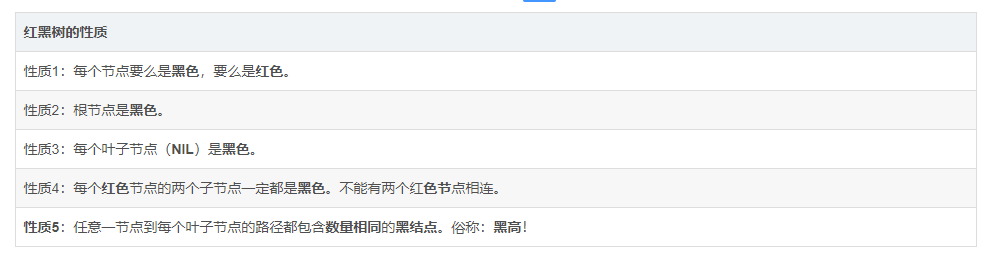static final class TreeNode<K,V> extends LinkedHashMap.Entry<K,V> {
TreeNode<K,V> parent;// 父节点
TreeNode<K,V> left;// 左子树
TreeNode<K,V> right;// 右子树
TreeNode<K,V> prev;    // needed to unlink next upon deletion
boolean red;// 颜色

/**
* 有参构造函数
*/
TreeNode(int hash, K key, V val, Node<K,V> next) {
super(hash, key, val, next);
}

/**
* 获取红黑树的根节点
*/
final TreeNode<K,V> root() {
for (TreeNode<K,V> r = this, p;;) {
if ((p = r.parent) == null)
return r;
r = p;
}
}

/**
* 确保给定的根root是树的第一个节点
*/
static <K,V> void moveRootToFront(Node<K,V>[] tab, TreeNode<K,V> root) {
...
}

/**
* 调用find方法查找.
*/
final TreeNode<K, V> getTreeNode(int h, Object k) {
// 从树的根节点开始查找
return ((parent != null) ? root() : this).find(h, k, null);
}

/**
* 从根节点出发查找具有给定哈希值和键的节点.从根节点出发
* 查找当前要插入节点node的父节点
*
* 经典二叉查找树的查找过程，先根据hash值比较，再根据key值比较决定是查左子树还是右子树。
*/
final TreeNode<K, V> find(int h, Object k, Class<?> kc) {
TreeNode<K, V> p = this;
do {
int ph, dir;
K pk;
TreeNode<K, V> pl = p.left, pr = p.right, q;
if ((ph = p.hash) > h)
// 左子树
p = pl;
else if (ph < h)
// 右子树
p = pr;
else if ((pk = p.key) == k || (k != null && k.equals(pk)))
// 找到了直接返回
return p;
else if (pl == null)
// hash相同但key不同，左子树为空查右子树
p = pr;
else if (pr == null)
// 右子树为空查左子树
p = pl;
else if ((kc != null ||
(kc = comparableClassFor(k)) != null) &&
(dir = compareComparables(kc, k, pk)) != 0)
// 通过compare方法比较key值的大小决定使用左子树还是右子树
p = (dir < 0) ? pl : pr;
else if ((q = pr.find(h, k, kc)) != null)
// 如果以上条件都不通过，则尝试在右子树查找
return q;
else
// 都没找到就在左子树查找
p = pl;
} while (p != null);
return null;
}

/**
* 用于在a 和 b 的hash值相等且不可比较时对插入进行排序
*/
static int tieBreakOrder(Object a, Object b) {
...
}

/**
* 对链表进行树化的方法
*（1）从链表的第一个元素开始遍历；
*（2）将第一个元素作为根节点；
*（3）其它元素依次插入到红黑树中，再做平衡；
*（4）将根节点移到链表第一元素的位置（因为平衡的时候根节点会改变）；
*/
final void treeify(Node<K, V>[] tab) {
TreeNode<K, V> root = null;
for (TreeNode<K, V> x = this, next; x != null; x = next) {
next = (TreeNode<K, V>) x.next;
x.left = x.right = null;
// 第一个元素作为根节点且为黑节点，其它元素依次插入到树中再做平衡
if (root == null) {
x.parent = null;
x.red = false;
root = x;
} else {
K k = x.key;
int h = x.hash;
Class<?> kc = null;
// 从根节点查找元素插入的位置
for (TreeNode<K, V> p = root; ; ) {
int dir, ph;
K pk = p.key;
if ((ph = p.hash) > h)
dir = -1;
else if (ph < h)
dir = 1;
else if ((kc == null &&
(kc = comparableClassFor(k)) == null) ||
(dir = compareComparables(kc, k, pk)) == 0)
dir = tieBreakOrder(k, pk);

// 如果最后没找到元素，则插入
TreeNode<K, V> xp = p;
if ((p = (dir <= 0) ? p.left : p.right) == null) {
x.parent = xp;
if (dir <= 0)
xp.left = x;
else
xp.right = x;
// 插入后平衡，默认插入的是红节点，在balanceInsertion()方法里
root = balanceInsertion(root, x);
break;
}
}
}
}
// 把根节点移动到链表的头节点，因为经过平衡之后原来的第一个元素不一定是根节点了
moveRootToFront(tab, root);
}

/**
* 对红黑树进行反树化的方法
*/
final Node<K,V> untreeify(HashMap<K,V> map) {
Node<K,V> hd = null, tl = null;
for (Node<K,V> q = this; q != null; q = q.next) {
Node<K,V> p = map.replacementNode(q, null);
if (tl == null)
hd = p;
else
tl.next = p;
tl = p;
}
return hd;
}

/**
* 向树种插入数据的方法
*（1）寻找根节点；
*（2）从根节点开始查找；
*（3）比较hash值及key值，如果都相同，直接返回，在putVal()方法中决定是否要替换value值；
*（4）根据hash值及key值确定在树的左子树还是右子树查找，找到了直接返回；
*（5）如果最后没有找到则在树的相应位置插入元素，并做平衡；
*/
final TreeNode<K, V> putTreeVal(HashMap<K, V> map, Node<K, V>[] tab,
int h, K k, V v) {
Class<?> kc = null;
// 标记是否找到这个key的节点
boolean searched = false;
// 找到树的根节点
TreeNode<K, V> root = (parent != null) ? root() : this;
// 从树的根节点开始遍历
for (TreeNode<K, V> p = root; ; ) {
// dir=direction，标记是在左边还是右边
// ph=p.hash，当前节点的hash值
int dir, ph;
// pk=p.key，当前节点的key值
K pk;
if ((ph = p.hash) > h) {
// 当前hash比目标hash大，说明在左边
dir = -1;
}
else if (ph < h)
// 当前hash比目标hash小，说明在右边
dir = 1;
else if ((pk = p.key) == k || (k != null && k.equals(pk)))
// 两者hash相同且key相等，说明找到了节点，直接返回该节点
// 回到putVal()中判断是否需要修改其value值
return p;
else if ((kc == null &&
// 如果k是Comparable的子类则返回其真实的类，否则返回null
(kc = comparableClassFor(k)) == null) ||
// 如果k和pk不是同样的类型则返回0，否则返回两者比较的结果
(dir = compareComparables(kc, k, pk)) == 0) {
// 这个条件表示两者hash相同但是其中一个不是Comparable类型或者两者类型不同
// 比如key是Object类型，这时可以传String也可以传Integer，两者hash值可能相同
// 在红黑树中把同样hash值的元素存储在同一颗子树，这里相当于找到了这颗子树的顶点
// 从这个顶点分别遍历其左右子树去寻找有没有跟待插入的key相同的元素
if (!searched) {
TreeNode<K, V> q, ch;
searched = true;
// 遍历左右子树找到了直接返回
if (((ch = p.left) != null &&
(q = ch.find(h, k, kc)) != null) ||
((ch = p.right) != null &&
(q = ch.find(h, k, kc)) != null))
return q;
}
// 如果两者类型相同，再根据它们的内存地址计算hash值进行比较
dir = tieBreakOrder(k, pk);
}

TreeNode<K, V> xp = p;
if ((p = (dir <= 0) ? p.left : p.right) == null) {
// 如果最后确实没找到对应key的元素，则新建一个节点
Node<K, V> xpn = xp.next;
TreeNode<K, V> x = map.newTreeNode(h, k, v, xpn);
if (dir <= 0)
xp.left = x;
else
xp.right = x;
xp.next = x;
x.parent = x.prev = xp;
if (xpn != null)
((TreeNode<K, V>) xpn).prev = x;
// 插入树节点后平衡
// 把root节点移动到链表的第一个节点
moveRootToFront(tab, balanceInsertion(root, x));
return null;
}
}
}

// remove 调用 removeNode
//public V remove(Object key) {
//    Node<K, V> e;
//    return (e = removeNode(hash(key), key, null, false, true)) == null ?
//            null : e.value;
//}

final Node<K, V> removeNode(int hash, Object key, Object value,
boolean matchValue, boolean movable) {
Node<K, V>[] tab;
Node<K, V> p;
int n, index;
// 如果桶的数量大于0且待删除的元素所在的桶的第一个元素不为空
if ((tab = table) != null && (n = tab.length) > 0 &&
(p = tab[index = (n - 1) & hash]) != null) {
Node<K, V> node = null, e;
K k;
V v;
if (p.hash == hash &&
((k = p.key) == key || (key != null && key.equals(k))))
// 如果第一个元素正好就是要找的元素，赋值给node变量后续删除使用
node = p;
else if ((e = p.next) != null) {
if (p instanceof TreeNode)
// 如果第一个元素是树节点，则以树的方式查找节点
node = ((TreeNode<K, V>) p).getTreeNode(hash, key);
else {
// 否则遍历整个链表查找元素
do {
if (e.hash == hash &&
((k = e.key) == key ||
(key != null && key.equals(k)))) {
node = e;
break;
}
p = e;
} while ((e = e.next) != null);
}
}
// 如果找到了元素，则看参数是否需要匹配value值，如果不需要匹配直接删除，
// 如果需要匹配则看value值是否与传入的value相等
if (node != null && (!matchValue || (v = node.value) == value ||
(value != null && value.equals(v)))) {
if (node instanceof TreeNode)
// 如果是树节点，调用树的删除方法（以node调用的，是删除自己）
((TreeNode<K, V>) node).removeTreeNode(this, tab, movable);
else if (node == p)
// 如果待删除的元素是第一个元素，则把第二个元素移到第一的位置
tab[index] = node.next;
else
// 否则删除node节点
p.next = node.next;
++modCount;
--size;
// 删除节点后置处理
afterNodeRemoval(node);
return node;
}
}
return null;
}

/**
*（1）先查找元素所在的节点；
*（2）如果找到的节点是树节点，则按树的移除节点处理；
*（3）如果找到的节点是桶中的第一个节点，则把第二个节点移到第一的位置；
*（4）否则按链表删除节点处理；
*（5）修改size，调用移除节点后置处理等；
*/
final void removeTreeNode(HashMap<K, V> map, Node<K, V>[] tab,
boolean movable) {
int n;
// 如果桶的数量为0直接返回
if (tab == null || (n = tab.length) == 0)
return;
// 节点在桶中的索引
int index = (n - 1) & hash;
// 第一个节点，根节点，根左子节点
TreeNode<K, V> first = (TreeNode<K, V>) tab[index], root = first, rl;
// 后继节点，前置节点
TreeNode<K, V> succ = (TreeNode<K, V>) next, pred = prev;

if (pred == null)
// 如果前置节点为空，说明当前节点是根节点，则把后继节点赋值到第一个节点的位置，相当于删除了当前节点
tab[index] = first = succ;
else
// 否则把前置节点的下个节点设置为当前节点的后继节点，相当于删除了当前节点
pred.next = succ;

// 如果后继节点不为空，则让后继节点的前置节点指向当前节点的前置节点，相当于删除了当前节点
if (succ != null)
succ.prev = pred;

// 如果第一个节点为空，说明没有后继节点了，直接返回
if (first == null)
return;

// 如果根节点的父节点不为空，则重新查找父节点
if (root.parent != null)
root = root.root();

// 如果根节点为空，则需要反树化（将树转化为链表）
// 如果需要移动节点且树的高度比较小，则需要反树化
if (root == null
|| (movable
&& (root.right == null
|| (rl = root.left) == null
|| rl.left == null))) {
tab[index] = first.untreeify(map);  // too small
return;
}

// 分割线，以上都是删除链表中的节点，下面才是直接删除红黑树的节点（因为TreeNode本身即是链表节点又是树节点）

// 删除红黑树节点的大致过程是寻找右子树中最小的节点放到删除节点的位置，然后做平衡，此处不过多注释
TreeNode<K, V> p = this, pl = left, pr = right, replacement;
if (pl != null && pr != null) {
TreeNode<K, V> s = pr, sl;
while ((sl = s.left) != null) // find successor
s = sl;
boolean c = s.red;
s.red = p.red;
p.red = c; // swap colors
TreeNode<K, V> sr = s.right;
TreeNode<K, V> pp = p.parent;
if (s == pr) { // p was s's direct parent
p.parent = s;
s.right = p;
} else {
TreeNode<K, V> sp = s.parent;
if ((p.parent = sp) != null) {
if (s == sp.left)
sp.left = p;
else
sp.right = p;
}
if ((s.right = pr) != null)
pr.parent = s;
}
p.left = null;
if ((p.right = sr) != null)
sr.parent = p;
if ((s.left = pl) != null)
pl.parent = s;
if ((s.parent = pp) == null)
root = s;
else if (p == pp.left)
pp.left = s;
else
pp.right = s;
if (sr != null)
replacement = sr;
else
replacement = p;
} else if (pl != null)
replacement = pl;
else if (pr != null)
replacement = pr;
else
replacement = p;
if (replacement != p) {
TreeNode<K, V> pp = replacement.parent = p.parent;
if (pp == null)
root = replacement;
else if (p == pp.left)
pp.left = replacement;
else
pp.right = replacement;
p.left = p.right = p.parent = null;
}

TreeNode<K, V> r = p.red ? root : balanceDeletion(root, replacement);

if (replacement == p) {  // detach
TreeNode<K, V> pp = p.parent;
p.parent = null;
if (pp != null) {
if (p == pp.left)
pp.left = null;
else if (p == pp.right)
pp.right = null;
}
}
if (movable)
moveRootToFront(tab, r);
}

/**
* Splits nodes in a tree bin into lower and upper tree bins,
* or untreeifies if now too small. Called only from resize;
* see above discussion about split bits and indices.
*
* @param map the map
* @param tab the table for recording bin heads
* @param index the index of the table being split
* @param bit the bit of hash to split on
*/
final void split(HashMap<K,V> map, Node<K,V>[] tab, int index, int bit) {
...
}

// 左旋
static <K,V> TreeNode<K,V> rotateLeft(TreeNode<K,V> root,
TreeNode<K,V> p) {
TreeNode<K,V> r, pp, rl;
if (p != null && (r = p.right) != null) {
if ((rl = p.right = r.left) != null)
rl.parent = p;
if ((pp = r.parent = p.parent) == null)
(root = r).red = false;
else if (pp.left == p)
pp.left = r;
else
pp.right = r;
r.left = p;
p.parent = r;
}
return root;
}

// 右旋
static <K,V> TreeNode<K,V> rotateRight(TreeNode<K,V> root,
TreeNode<K,V> p) {
TreeNode<K,V> l, pp, lr;
if (p != null && (l = p.left) != null) {
if ((lr = p.left = l.right) != null)
lr.parent = p;
if ((pp = l.parent = p.parent) == null)
(root = l).red = false;
else if (pp.right == p)
pp.right = l;
else
pp.left = l;
l.right = p;
p.parent = l;
}
return root;
}

// 修复红黑树平衡的方法
static <K,V> TreeNode<K,V> balanceInsertion(TreeNode<K,V> root,
TreeNode<K,V> x) {
x.red = true;
for (TreeNode<K,V> xp, xpp, xppl, xppr;;) {
if ((xp = x.parent) == null) {
x.red = false;
return x;
}
else if (!xp.red || (xpp = xp.parent) == null)
return root;
if (xp == (xppl = xpp.left)) {
if ((xppr = xpp.right) != null && xppr.red) {
xppr.red = false;
xp.red = false;
xpp.red = true;
x = xpp;
}
else {
if (x == xp.right) {
root = rotateLeft(root, x = xp);
xpp = (xp = x.parent) == null ? null : xp.parent;
}
if (xp != null) {
xp.red = false;
if (xpp != null) {
xpp.red = true;
root = rotateRight(root, xpp);
}
}
}
}
else {
if (xppl != null && xppl.red) {
xppl.red = false;
xp.red = false;
xpp.red = true;
x = xpp;
}
else {
if (x == xp.left) {
root = rotateRight(root, x = xp);
xpp = (xp = x.parent) == null ? null : xp.parent;
}
if (xp != null) {
xp.red = false;
if (xpp != null) {
xpp.red = true;
root = rotateLeft(root, xpp);
}
}
}
}
}
}

static <K,V> TreeNode<K,V> balanceDeletion(TreeNode<K,V> root,
TreeNode<K,V> x) {
for (TreeNode<K,V> xp, xpl, xpr;;) {
if (x == null || x == root)
return root;
else if ((xp = x.parent) == null) {
x.red = false;
return x;
}
else if (x.red) {
x.red = false;
return root;
}
else if ((xpl = xp.left) == x) {
if ((xpr = xp.right) != null && xpr.red) {
xpr.red = false;
xp.red = true;
root = rotateLeft(root, xp);
xpr = (xp = x.parent) == null ? null : xp.right;
}
if (xpr == null)
x = xp;
else {
TreeNode<K,V> sl = xpr.left, sr = xpr.right;
if ((sr == null || !sr.red) &&
(sl == null || !sl.red)) {
xpr.red = true;
x = xp;
}
else {
if (sr == null || !sr.red) {
if (sl != null)
sl.red = false;
xpr.red = true;
root = rotateRight(root, xpr);
xpr = (xp = x.parent) == null ?
null : xp.right;
}
if (xpr != null) {
xpr.red = (xp == null) ? false : xp.red;
if ((sr = xpr.right) != null)
sr.red = false;
}
if (xp != null) {
xp.red = false;
root = rotateLeft(root, xp);
}
x = root;
}
}
}
else { // symmetric
if (xpl != null && xpl.red) {
xpl.red = false;
xp.red = true;
root = rotateRight(root, xp);
xpl = (xp = x.parent) == null ? null : xp.left;
}
if (xpl == null)
x = xp;
else {
TreeNode<K,V> sl = xpl.left, sr = xpl.right;
if ((sl == null || !sl.red) &&
(sr == null || !sr.red)) {
xpl.red = true;
x = xp;
}
else {
if (sl == null || !sl.red) {
if (sr != null)
sr.red = false;
xpl.red = true;
root = rotateLeft(root, xpl);
xpl = (xp = x.parent) == null ?
null : xp.left;
}
if (xpl != null) {
xpl.red = (xp == null) ? false : xp.red;
if ((sl = xpl.left) != null)
sl.red = false;
}
if (xp != null) {
xp.red = false;
root = rotateRight(root, xp);
}
x = root;
}
}
}
}
}

/**
* Recursive invariant check
*/
static <K,V> boolean checkInvariants(TreeNode<K,V> t) {
TreeNode<K,V> tp = t.parent, tl = t.left, tr = t.right,
tb = t.prev, tn = (TreeNode<K,V>)t.next;
if (tb != null && tb.next != t)
return false;
if (tn != null && tn.prev != t)
return false;
if (tp != null && t != tp.left && t != tp.right)
return false;
if (tl != null && (tl.parent != t || tl.hash > t.hash))
return false;
if (tr != null && (tr.parent != t || tr.hash < t.hash))
return false;
if (t.red && tl != null && tl.red && tr != null && tr.red)
return false;
if (tl != null && !checkInvariants(tl))
return false;
if (tr != null && !checkInvariants(tr))
return false;
return true;
}
}

（1）TreeNode本身既是链表节点也是红黑树节点；

（2）先删除链表节点；

（3）再删除红黑树节点并做平衡；

5 将链表转换为红黑树 treeifyBin()

if (binCount >= TREEIFY_THRESHOLD - 1) // -1 for 1st
//转换为红黑树 tab表示数组名  hash表示哈希值
treeifyBin(tab, hash);

treeifyBin 方法如下所示：

/*
替换指定哈希表的索引处桶中的所有链接结点，除非表太小，否则将修改大小。
Node<K,V>[] tab = tab 数组名
int hash = hash表示哈希值
*/
final void treeifyBin(Node<K,V>[] tab, int hash) {
int n, index; Node<K,V> e;
/*
如果当前数组为空或者数组的长度小于进行树形化的阈值(MIN_TREEIFY_CAPACITY = 64)，
就去扩容。而不是将结点变为红黑树。
目的：如果数组很小，那么转换红黑树，然后遍历效率要低一些。这时进行扩容，
那么重新计算哈希值，链表长度有可能就变短了，数据会放到数组中，这样相对来说效率高一些。
*/
if (tab == null || (n = tab.length) < MIN_TREEIFY_CAPACITY)
//扩容方法
resize();
else if ((e = tab[index = (n - 1) & hash]) != null) {
/*
1）执行到这里说明哈希表中的数组长度大于阈值64，开始进行树形化
2）e = tab[index = (n - 1) & hash]表示将数组中的元素取出赋值给e，
e是哈希表中指定位置桶里的链表结点，从第一个开始
*/
// hd：红黑树的头结点   tl：红黑树的尾结点
TreeNode<K,V> hd = null, tl = null;
do {
// 新创建一个树的结点，内容和当前链表结点e一致
TreeNode<K,V> p = replacementTreeNode(e, null);
if (tl == null)
hd = p; // 将新创键的p结点赋值给红黑树的头结点
else {
p.prev = tl; // 将上一个结点p赋值给现在的p的前一个结点
tl.next = p; // 将现在结点p作为树的尾结点的下一个结点
}
tl = p;
/*
e = e.next 将当前结点的下一个结点赋值给e，如果下一个结点不等于null
则回到上面继续取出链表中结点转换为红黑树
*/
} while ((e = e.next) != null);
/*
让桶中的第一个元素即数组中的元素指向新建的红黑树的结点，以后这个桶里的元素就是红黑树
而不是链表数据结构了
*/
if ((tab[index] = hd) != null)
hd.treeify(tab);
}
}26793 010084 014498 010883 011818 0windows server 2008阿里云ECS服务器安全设置

9161 013890 04659 07365 022404 0

87

0

《2021云上架构与运维峰会演讲合集》

《零基础CSS入门教程》

《零基础HTML入门教程》